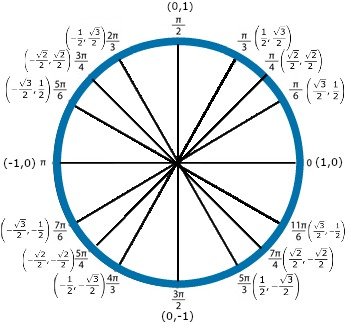# What is tan(0)?

What is tan(0)?

## The Unit Circle:

In trigonometry, the unit circle is a handy tool that we can use to evaluate trigonometric functions of various angles. This circle has its center at the origin of a graph, and a radius of length 1 unit. Each point on the unit circle has form (cos(θ), sin(θ)), where θ is the angle formed counterclockwise between the positive x-axis and the ray extending from the origin to that point on the circle.

tan(0) = 0

We can calculate this using a trigonometric identity and the unit circle. The identity we will be using is as follows:

• {eq}tan(\theta )=\frac{sin(\theta )}{cos(\theta )} {/eq}

On the unit circle, the angle θ = 0 corresponds to the point (1,0) as shown in the image.By the definition of the unit circle, we have the following:

• (1,0) = (cos(0), sin(0))

Therefore, we have that cos(0) = 1 and sin(0) = 0. Plugging these into our trigonometric identity for tan(0), we get the following:

• {eq}tan(0)=\frac{sin(0)}{cos(0)}=\frac{0}{1}=0 {/eq}

All together, this gives that tan(0) = 0.